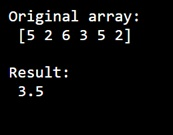# Calculating a rolling (weighted) average using numpy

Learn, how to calculate a rolling (weighted) average using numpy?
Submitted by Pranit Sharma, on December 31, 2022

NumPy is an abbreviated form of Numerical Python. It is used for different types of scientific operations in python. Numpy is a vast library in python which is used for almost every kind of scientific or mathematical operation. It is itself an array which is a collection of various methods and functions for processing the arrays.

## Calculating a rolling (weighted) average

we can compute the weighted of a given array with the help of numpy.average() function in which we pass the weight array in the parameter.

This method returns the weighted average of an array over the given axis. The weights array can either be 1-D (in which case its length must be the size of an along the given axis) or of the same shape as a. If weights=None, then all data in a are assumed to have a weight equal to one.

Let us understand with the help of an example,

## Python code to calculate a rolling (weighted) average using numpy

```# Import numpy
import numpy as np

# Creating two numpy array of 4x4
arr = np.array([5,2,6,3,5,2])

# Display original array
print("Original array:\n",arr,"\n")

# Calculate weighted average
res = np.average(arr, weights=[3, 1, 0, 0,2,4])

# Display result
print("Result:\n",res,"\n")
```

Output: